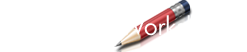Solve a quadratic equation in standard form. Solve for real as well as complex roots. Remember, standard form for a quadratic equation is as follows:

a x 2 + b x + c = 0

Enter the values of the coefficients of a quadratic equation:

 Value of a: Value of b: Value of c:

 First Root: Second Root: